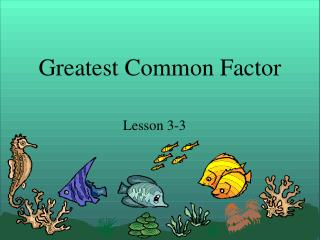# Greatest Common Factor - PowerPoint PPT PresentationDownload PresentationGreatest Common Factor

Greatest Common FactorDownload Presentation## Greatest Common Factor

- - - - - - - - - - - - - - - - - - - - - - - - - - - E N D - - - - - - - - - - - - - - - - - - - - - - - - - - -
##### Presentation Transcript

1. Greatest Common Factor Lesson 3-3

2. Greatest Common Factor (GCF) • The greatest common factor is the largest factor that two numbers share. • Let’s find the GCF of 12 and 42. First, we need to make a list of factors for each number.

3. 12 42 1 x 12 1 x 42 Factors of 12: 1, 2, 3, 4, 6,12 2 x 21 2 x 6 3 x 14 3 x 4 4 x ?? 4 x 3 Factors of 42: 1, 2, 3, 6, 7, 14, 21, 42 5 x ?? 6 x 7 7 x 6 Common Factors: 1, 2, 3, 6 Greatest Common Factor: 6

4. What is the GCF of 18 and 27? 18 27 Factors of 18: 1, 2, 3, 6, 9, 18 1 x 18 1 x 27 2 x ? 2 x 9 3 x 9 3 x 6 Factors of 27: 1, 3, 9, 27 4 x ? 5 x ? 4 x ? 6 x ? 5 x ? 7 x ? Common Factors: 1, 3, 9 8 x ? 6 x 3 9 x 3 GCF: 9

5. What is the GCF of 48 and 60? Factors of 48: 1, 2, 3, 4, 6, 8, 12, 16, 24, 48 48 60 1 x 48 1 x 60 2 x 30 2 x 24 3 x 20 3 x 16 4 x 15 Factors of 60: 1, 2, 3, 4, 5, 6, 10, 12, 15, 20, 30, 60 4 x 12 5 x 12 6 x 8 6 x 10 Common Factors: 1, 2, 3, 4, 6, 12 GCF: 12

6. Example: GCF of 27 and 36 27 36 • Both prime factorizations have 3x3 in common. • Therefore, the GCF is 9. 2 x 18 3 x 9 2 x 9 3 x 3 3 x 3 Ans. 3x3x3 Ans. 2x2x3x3

7. Common Multiple • A number that is a multiple of two or more numbers. Some multiples of 3 & 4 12, 24, 36, 48,

8. Least Common Multiple • The smallest common multiple of a set of two or more numbers. 4 = 4, 8, 12, 16, 20, 24, 28 5 = 5, 10, 15, 20, 25, 30

9. Homework Time